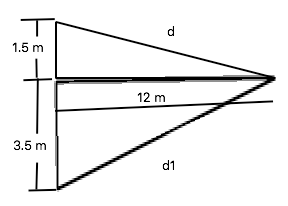# Two-in-phase loudspeakers that emit sound with the same frequency are placed along a wall and are...

## Question:

Two-in-phase loudspeakers that emit sound with the same frequency are placed along a wall and are separated by a distance of {eq}5.00\ m {/eq}. A person is standing {eq}12.0\ m {/eq} away from the wall, equidistant from the loudspeakers. When the person moves {eq}1.00\ m {/eq} parallel to the wall, she experiences destructive interference for the first time. What is the frequency of the sound? The speed of sound in air is {eq}343\ \dfrac ms {/eq}.

A. 211 Hz.

b. 256 Hz.

C. 422 Hz.

D. 512 Hz.

E. 674 Hz.

## Interference

Diffraction is the capacity of a wave to bend around the edge of an obstacle. while interference is the combination of two or more waves to form a resultant wave in which the displacement is either reinforced (constructive interference) or canceled (destructive interference).

The Condition for destructive interference is,

{eq}d' - d = (m + \dfrac 12 \lambda ) {/eq}

Wherein,

{eq}\lambda {/eq} is the wavelength of the wave and d is the separation distance.

## Answer and Explanation:

The below figure indicates the destructive interference from the given data:Determining the distance "d" from the top triangle in the figure above,

{eq}d = \sqrt {(1.5m)^2 + (12 m)^2 } \ = \ 12.09 \ m {/eq}

Determining the distance "d1" from the bottom triangle in the figure above,

{eq}d1 = \sqrt {(3.5m)^2 + (12 m)^2 } \ = \ 12.5 \ m {/eq}

Since, she experiences teh destructive interference for the first time, the value of m there is zero.

{eq}d1 - d = (0 + \dfrac 12 \lambda ) \\ 12.5 - 12.093386 = \dfrac 12 \lambda \\ \lambda = 2 (0.406614 m) \\ \lambda = 0.813228 \ m {/eq}

The frequency of sound wave is determined as,

{eq}f \ = \ \dfrac {343 \dfrac ms}{0.813228 \ m } \\ f \ = \ 421.77 \ Hz \\ \\ f \ = \ 422 \ Hz {/eq}

The answer is (C) 422 Hz.